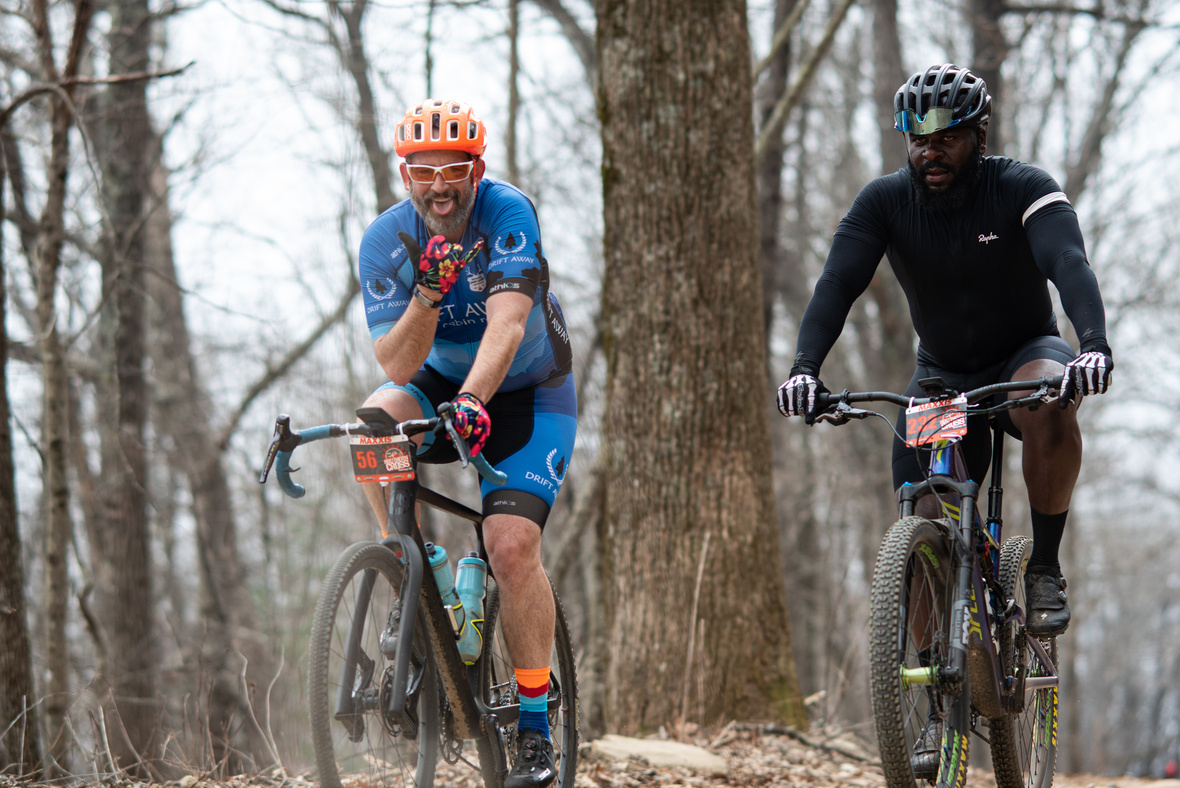table.module-1{width:99.25%;padding:0}table div table+table div table{width:99.25%;float:none;margin-left:auto;margin-right:auto;padding:0}table div table+table div table a{border:0 none;text-decoration:none}table div table+table div table img{width:100%!important;border:0 none;text-decoration:none}table div table+table div table td{width:100%;padding:0}/* styles */

## RACE DETAILS! Maxxis Tires Southern Cross Bike Race!# Thank you for signing up for the 2023 Maxxis Tires Southern Cross Bike Race!

We have another sellout crowd this year and we are thrilled that you all are excited to join us for this event! Please read the comprehensive PARTICIPANT GUIDE below, as it provides every detail possible about the upcoming race.

Please click through all the buttons below - you have THREE things to do before Monday Feb 27th...

CHECK YOUR NAME AND CATEGORY on the Participant List - if you want to change categories, you must contact me before Feb 27th. You cannot change categories during/after the race. Please note the Mens Expert/Open and Womens Expert/Open are the "money" classes, so you need to be registered in and start in either of those to be eligible for the bigger cash awards.Just to reiterate - click through all these links. Any category changes or rider transfers need to be done by February 27th.

If you can no longer attend, all transfers need to be done by February 27th at the absolute latest. If you want to sell your entry, you are responsible for finding that person and working out payment with them. All I need is an email from YOU approving the transfer, and the new rider's name and email so I can reach out and get them registered...and all this needs to happen BEFORE FEBRUARY 27th.

Thank you for signing up and we look forward to seeing everyone in March!

Lisa Randalltable div table+table+table+table+table+table+table+table+table+table div table{width:100%;padding:0}table div table+table+table+table+table+table+table+table+table+table div table img{width:96.23%;padding:0;float:none}table div table+table+table+table+table+table+table+table+table+table div table td{width:100%;padding:0 1.88% 18px}/* styles */## Torq Fueled Southern Cross - 25% Discount for all Racers!

We are excited to have Torq Energy and Torq Gels on course at this year's Southern Cross! Get you fuel dialed in now with TORQ USA!

Read TORQ USA’s latest article on best hydration practices HERE.

# 25% Discount Code for ALL PARTICIPANTS: SOUTHERNCX2023

 table.module-12{width:98.11%;padding:0}table div table+table+table+table+table+table+table+table+table+table+table+table+table div table{width:98.11%;float:none;margin-left:auto;margin-right:auto;padding:0}table div table+table+table+table+table+table+table+table+table+table+table+table+table div table a{border:0 none;text-decoration:none}table div table+table+table+table+table+table+table+table+table+table+table+table+table div table img{width:100%!important;border:0 none;text-decoration:none}table div table+table+table+table+table+table+table+table+table+table+table+table+table div table td{width:100%;padding:0}/* styles */
 table div table+table+table+table+table+table+table+table+table+table+table+table+table+table div table{width:100%;padding:0}table div table+table+table+table+table+table+table+table+table+table+table+table+table+table div table img{width:96.23%;padding:0;float:none}table div table+table+table+table+table+table+table+table+table+table+table+table+table+table div table td{width:100%;padding:0 1.88% 18px}/* styles */table div table+table+table+table+table+table+table+table+table+table+table+table+table+table+table div table,table.module-14{width:41.89%;float:left;padding:0}table div table+table+table+table+table+table+table+table+table+table+table+table+table+table+table div table a{border:0 none;text-decoration:none}table div table+table+table+table+table+table+table+table+table+table+table+table+table+table+table div table img{width:100%!important;border:0 none;text-decoration:none}table div table+table+table+table+table+table+table+table+table+table+table+table+table+table+table div table td{width:100%;padding:0 20px 20px 0}/* styles */ If you are looking for an elegant dinner or date night on Friday or Saturday night while at the race, check out The Restaurant at Montaluce. The entrees and desserts are amazing - you will not be disappointed! Reservations are required as it is a small restaurant. Please let them know you are in town for the race if you make a dinner reservation. If you are looking for more casual lunch or dinner fare, Montaluce has a new restaurant called Trattoria di Montaluce which offers Italian Cuisine served family style as well as specialty pizzas. If you have friends and family joining you for the race, Montaluce also offers several experiences such as Wine Tastings, Wine Hikes and Fly Fishing to keep your guests entertained while you race.
 table div table+table+table+table+table+table+table+table+table+table+table+table+table+table+table+table div table{width:100%;padding:0}table div table+table+table+table+table+table+table+table+table+table+table+table+table+table+table+table div table img{width:96.23%;padding:0;float:none}table div table+table+table+table+table+table+table+table+table+table+table+table+table+table+table+table div table td{width:100%;padding:0 1.88% 18px}/* styles */## Come Back and Visit Mulberry Gap Adventure Basecamp!

 table div table+table+table+table+table+table+table+table+table+table+table+table+table+table+table+table+table+table div table td,table.module-17{width:100%;padding:0}table div table+table+table+table+table+table+table+table+table+table+table+table+table+table+table+table+table+table div table{width:100%;float:none;margin-left:auto;margin-right:auto;padding:0}table div table+table+table+table+table+table+table+table+table+table+table+table+table+table+table+table+table+table div table a{border:0 none;text-decoration:none}table div table+table+table+table+table+table+table+table+table+table+table+table+table+table+table+table+table+table div table img{width:100%!important;border:0 none;text-decoration:none}/* styles */
 table div table+table+table+table+table+table+table+table+table+table+table+table+table+table+table+table+table+table+table div table td,table.module-18{width:100%;padding:0}table div table+table+table+table+table+table+table+table+table+table+table+table+table+table+table+table+table+table+table div table{width:100%;float:none;margin-left:auto;margin-right:auto;padding:0}table div table+table+table+table+table+table+table+table+table+table+table+table+table+table+table+table+table+table+table div table a{border:0 none;text-decoration:none}table div table+table+table+table+table+table+table+table+table+table+table+table+table+table+table+table+table+table+table div table img{width:100%!important;border:0 none;text-decoration:none}/* styles */
 table div table+table+table+table+table+table+table+table+table+table+table+table+table+table+table+table+table+table+table+table div table{width:100%;padding:0}table div table+table+table+table+table+table+table+table+table+table+table+table+table+table+table+table+table+table+table+table div table img{width:96.23%;padding:0;float:none}table div table+table+table+table+table+table+table+table+table+table+table+table+table+table+table+table+table+table+table+table div table td{width:100%;padding:0 1.88% 18px}/* styles */table div table+table+table+table+table+table+table+table+table+table+table+table+table+table+table+table+table+table+table+table+table+table div table{width:100%;padding:0}table div table+table+table+table+table+table+table+table+table+table+table+table+table+table+table+table+table+table+table+table+table+table div table img{width:96.23%;padding:0;float:none}table div table+table+table+table+table+table+table+table+table+table+table+table+table+table+table+table+table+table+table+table+table+table div table td{width:100%;padding:0 1.88% 18px}/* styles */## 2023 Full Event Calendar

 /* styles */ Want to check out our other events? Our 2023 calendar is below - we focus on mountain bike races, trail runs, and off-road duathlons. March 4, 2023 - Southern Cross 50 Mile Gravel Grind March 18, 2023 - Assault on Garland Mountain 10k and 13.1 mile Trail Run April 1, 2023 - Blankets Creek Dirty 15/30 Mountain Bike Race April 1, 2023 - Blankets Creek 5 and 9 mile Trail Run April 23, 2023 - Big Frog 50k - 13.1 mile - 7 mile Trail Runs May 27, 2023 - Rope Mill 10k and 13.1 mile Trail Run May 27, 2023 - Rope Mill Fast+Furious 15 MTB Race September 9, 2023 - Fools Gold 38 and 60 Mile MTB Races September 30, 2023 - Blankets Creek 3/6 Hour Race November 11, 2023 - Rope Mill Dirty Duathlon and 5k Trail Run Stay on top of Southern Cross sponsor and event announcements by liking the Southern Cross Facebook page. While you're at it, we'd love for you to like the Mountain Goat Adventures page as well!table div table+table+table+table+table+table+table+table+table+table+table+table+table+table+table+table+table+table+table+table+table+table+table+table+table+table div table{width:100%;padding:0}table div table+table+table+table+table+table+table+table+table+table+table+table+table+table+table+table+table+table+table+table+table+table+table+table+table+table div table img{width:96.23%;padding:0;float:none}table div table+table+table+table+table+table+table+table+table+table+table+table+table+table+table+table+table+table+table+table+table+table+table+table+table+table div table td{width:100%;padding:0 1.88% 18px}/* styles */